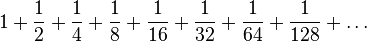# Series facts for kids

Kids Encyclopedia Facts

A series is a group of several things that are all about the same thing, or are intentionally similar.

In mathematics, a series is the sum of a never ending mathematical sequence (a list of numbers). For example:$1 + \frac12 + \frac14 + \frac18 + \frac1{16} + \frac1{32} + \frac1{64} + \frac1{128} + \ldots$

The dots mean that the sum does not have a last term, but goes on to infinity.Series Facts for Kids. Kiddle Encyclopedia.